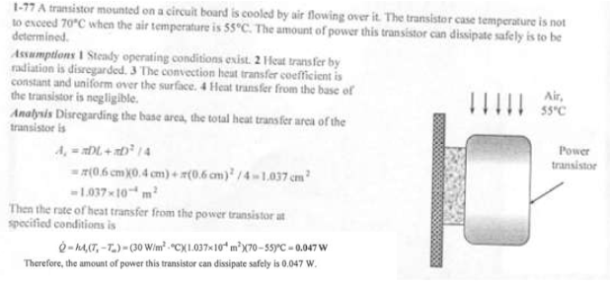heat and mass transfer problems engineering equations heat and mass transfer school homework engineering heat and mass transfer formulas heat and mass transfer solutions to heat and mass transfer problems full solution engineering problem solution heat and mass transfer math problems engineering equations heat and mass transfer school homework engineering solutions to heat and mass transfer formulas heat problems mass problem solutions to transfer problems full solution heat and mass transfer
heat and mass transfer problems engineering equations heat and mass transfer school homework engineering heat and mass transfer formulas heat and mass transfer solutions to heat and mass transfer problems full solution engineering problem solution heat and mass transfer math problems engineering equations heat and mass transfer school homework engineering solutions to heat and mass transfer formulas heat problems mass problem solutions to transfer problems full solution heat and mass transfer
Highalphabet Home Page heat and mass transfer problem solutions Heat and Mass Transfer Page
A transistor with a height of 0.4 cm and a diameter of 0.6 cm is mounted on a circuit board. The transistor is cooled by air flowing over it with an average heat transfer coefficient of 30 W/m^2K. If the air temperature is 55 C and the transistor case temperature is not to exceed 70 C, determine the amount of power this transistor can dissipate safely. Disregard any heat transfer from the transistor base.A transistor with a height of 0.4 cm and a diameter of 0.6 cm is mounted on a circuit board. The transistor is cooled by air flowing over it with an average heat transfer coefficient of 30 W/m^2K. If the air temperature is 55 C and the transistor case temperature is not to exceed 70 C, determine the amount of power this transistor can dissipate safely. Disregard any heat transfer from the transistor base.